# SAFETY CONCEPT IN STRUCTURAL STEEL DESIGNReading time: 1 minute

SAFETY CONCEPT IN STRUCTURAL STEEL DESIGN

There will be a gap between the internal load and the internal resistance in the members of the structure. The gap is covered in either of the two ways:

a) In elastic design the allowable stresses are taken less than the strength of the material by an appropriate factor called factor of safety.

b) In ultimate strength design, the internal load is increased by a factor called the load factor, whereas the ultimate strength is computed on the basis of yield or buckling strength.

Let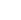be the internal load and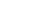be the increase in load. Letbe the internal strength andbe the possible under-strength, then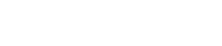This expression indicates that the computed strength should be equal to the internal load multiplied by a factor greater than one. This is the ultimate load analysis where in the full strength is considered and loads are increased by a load factor.

AlsoThis is the elastic design approach, and the dividing factor is called the factor of safety.

FACTOR OF SAFETY

In the elastic design of steel structures, the factor of safety is applied on the yield stress of the material to obtain the working stress or permissible stress in the material.

The value of factor of safety is decided considering the followings:

1. The average strength of materials is determined after making tests on number of specimens. The strengths of different specimens of given structural material are not identical.
2. The values of design loads remain uncertain, but values of dead loads can be determined correctly. But live load, impact load, wind load, snow load, etc cannot be determined with certainty since these depend upon statistics available. The probable values of these loads are only determined.
3. The values of internal forces in many structures depend upon the methods of analysis. The degree of precision of different methods varies. The methods involving detailed analysis are more precise. In case, analysis of the structure is done precisely, a small value of factor of safety may be adopted.
4. During fabrication, structural steel is subjected to different operations. The punching of a hole in a structural element distorts the surrounding material and cause high residual stresses. The warping and buckling of elements may take place during welding. The welding leaves high residual stresses. Structural elements are subjected to uncertain stress.
5. The variations in temperatures and settlement of supports are uncertain. Many times, a well designed structure is damaged because of these effects. The strength of materials decreases because of corrosion. The extent of corrosion is more when a structure is located in industrial areas and exposed to chemical wastes.
6. The failure of some structures or some elements of a structure is less serious and less disastrous than the failure of large structures or a main element of a structure.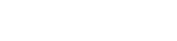The load factor depends upon the nature of loading, the support conditions and the geometrical shape of the structural member. The bending moment at any section is directly proportional to the applied load. Therefore, bending moment M is proportional to W.

or M = kW

The fully plastic moment is also directly proportional to the collapse load,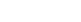If the maximum bending moment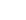corresponds to the maximum working load, then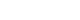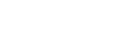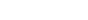where Z = elastic modulus

f = working stress or permissible stress.

Again,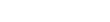Where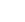= plastic modulus, and= yield stress of steel.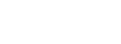or, load factor Q = S x F

Where S is the shape factor =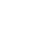, and

F = factor of safety used in the elastic design.

In general, for gravity loads, the value of load factor adopted is 1.85. where wind loads are considered, the permissible stress is increased by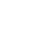percent. This reduces the load factor to 1.4 for wind loads.